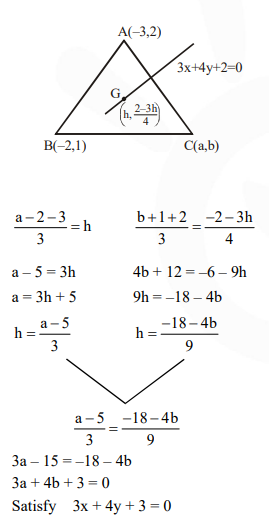# Solve the equation

Question:

Let $A(-3,2)$ and $B(-2,1)$ be the vertices of a triangle $A B C$. If the centroid of this triangle lies on the line $3 x+4 y+2=0$, then the vertex $\mathrm{C}$ lies on the line :

1.  4x + 3y + 5 = 0

2. 3x + 4y + 5 = 0

3.  3x + 4y + 3 = 0

4. 4x + 3y + 3 = 0

Correct Option: , 3

Solution: